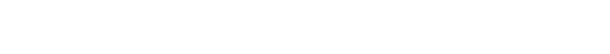Q矩阵初始化的时候全为0，因为它的状态我们已经全部知道了，所以我们知道总的状态是6。如果我们并不知道有多少个状态，那幺请从1个状态开始，一旦发现新的状态，那幺为这个矩阵添加上新的行和列。

Q(state, action) = R(state, action) + Gamma * Max[Q(next state, all actions)]

Q-learning算法如下：

1 设置gamma相关系数，以及奖励矩阵R

2 将Q矩阵初始化为全0

3 For each episode：

Do While 当没有到达目标时?

End Do

End ForQ(state, action) = R(state, action) + Gamma * Max[Q(next state, all actions)]

Q(1, 5) = R(1, 5) + 0.8 * Max[Q(5, 1), Q(5, 4), Q(5, 5)]= 100 + 0.8 * 0 = 100Q(state, action) = R(state, action) + Gamma * Max[Q(next state, all actions)]

Q(3, 1) = R(3, 1) + 0.8 * Max[Q(1, 2), Q(1, 5)]= 0 + 0.8 * Max(0, 100) = 80Q(state, action) = R(state, action) + Gamma * Max[Q(next state, all actions)]

Q(1, 5) = R(1, 5) + 0.8 * Max[Q(1, 2), Q(1, 5)]= 0 + 0.8 * Max(0, 100) = 80